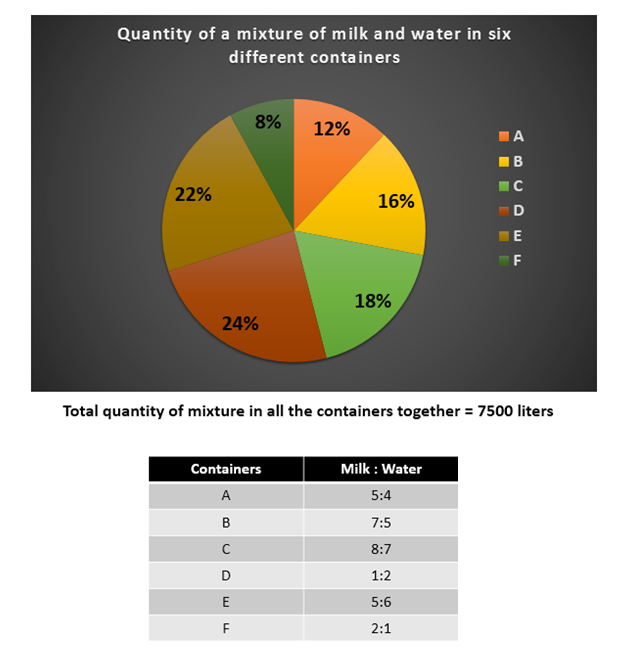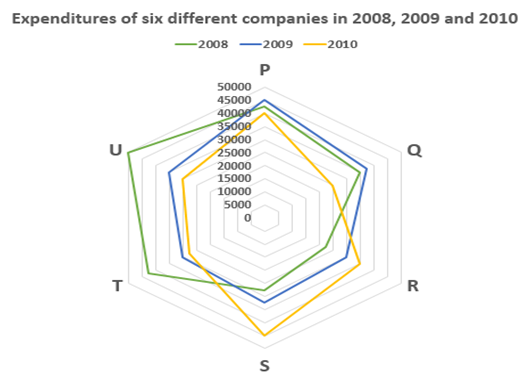# Quantitative Aptitude Questions (Data Interpretation) for SBI Clerk / IDBI Executive 2018 Day-48

Dear Readers, SBI is conducting Online preliminary Examination for the recruitment of Clerical Cadre. preliminary Examination of SBI Clerk was scheduled from June/July 2018. To enrich your preparation here we have providing new series of Data Interpretation – Quantitative Aptitude Questions. Candidates those who are appearing in SBI Clerk Prelims and IDBI Executive Exams can practice these Quantitative Aptitude average questions daily and make your preparation effective.

Click “Start Quiz” to attend these Questions and view Solutions

Directions (Q. 1 – 5): Study the following information carefully and answer the questions given below:1. Container G also contains mixture of milk and water. If the quantity of milk in G is 15% more than that in F and quantity of water in G is 10% more than that in E. Find the respective ratio of milk and water in container G.

1. 99:46
2. 45:89
3. 46:99
4. 89:45
5. None of these
1. Find the difference between quantity of milk in A and B together and quantity of water in C and D together.
1. 540 litres
2. 490 litres
3. 630 litres
4. 350 litres
5. None of these
1. Quantity of water in B, C and D together is what percent of quantity of water in A, E and F together?
1. 133.55%
2. 144.66%
3. 166.44%
4. 155.33%
5. None of these
1. Find the quantity of milk in all the containers together.
1. 3670 litres
2. 4250 litres
3. 3760 litres
4. 4520 litres
5. none of these
1. If 20 litres extra milk and 30 litres extra water is added to container D. Mixture in container D forms what percent of total quantity of mixture in all the containers together?
1. 23.4%
2. 24.5%
3. 26.7%
4. 27.8%
5. None of these

Directions (Q. 6 – 10): Study the following information carefully and answer the questions given below:1. Expenditures of companies P and Q together in 2008 is what percent of the expenditures of companies T and U in 2009?
1. 116.23%
2. 105.32%
3. 119.23%
4. 117.23%
5. None of these
1. What is the difference between expenditures of all the companies in 2009 and 2010?
1. Rs.7000
2. Rs.7500
3. Rs.8500
4. Rs.8000
5. None of these
1. Find the respective ratio of sum of expenditures of companies P, Q and R in 2009 and that of companies S, T and U in 2010.
1. 35:31
2. 45:41
3. 31:35
4. 41:45
5. None of these
1. Expenditures of companies S and T together in 2008 is what percent less than the expenditures of companies R and S together in 2010?
1. 10.5%
2. 11.5%
3. 12.5%
4. 14.14%
5. None of these
1. If the profit percent of company R in 2009 is 25% and that of company T in 2010 is 18%, find the sum of their incomes.
1. Rs.67940
2. Rs.69850
3. Rs.69950
4. Rs.67950
5. None of these

Directions (Q. 1 – 5):

Quantity of milk in F = 2/3 x 8/100 x 7500 = 400 litres

Quantity of milk in G = 400 x 115/100 = 460 litres

Quantity of water in E = 6/11 x 22/100 x 7500 = 900 litres

Quantity of water in G = 900 x 110/100 = 990 litres

Required ratio = 460 : 990 = 46 : 99

Quantity of milk in A = 5/9 x 12/100 x 7500 = 500 litres

Quantity of milk in B = 7/12 x 16/100 x 7500 = 700 litres

Quantity of water in C = 7/15 x 18/100 x 7500 = 630 litres

Quantity of water in D = 2/3 x 24/100 x 7500 = 1200 litres

Required difference = (1200 + 630) – (500 + 700)

= 1830 – 1200

= 630 litres

Quantity of water in B, C and D together

= 5/12 x 16/100 x 7500 + 7/15 x 18/100 x 7500 + 2/3 x 24/100 x 7500

= 500 + 630 + 1200

= 2330 litres

Quantity of water in A, E and F together

= 4/9 x 12/100 x 7500 + 6/11 x 22/100 x 7500 + 1/3 x 8/100 x 7500

= 400 + 900 + 200

= 1500 litres

Required percentage = (2330/1500) x 100 = 155.33%

Quantity of milk in A = 5/9 x 12/ 100 x 7500 = 500 litres

Quantity of milk in B = 7/12 x 16/100 x 7500 = 700 litres

Quantity of milk in C = 8/15 x 18/100 x 7500 = 720 litres

Quantity of milk in D = 1/3 x 24/100 x 7500 = 600 litres

Quantity of milk in E = 5/11 x 22/100 x 7500 = 750 litres

Quantity of milk in F = 2/3 x 8/100 x 7500 = 400 litres

Required sum = 500 + 700 + 720 + 600 + 750 + 400 = 3670 litres

Quantity of mixture in container D = 24/100 x 7500 = 1800 litres

New quantity of mixture in container D = (1800 + 20 + 30) = 1850 litres

Required percentage = [1850 / (7500 + 20 + 30)] x 100

= [1850/7550] x 100

= 24.5%

Directions (Q. 6 – 10):

Expenditures of P and Q together in 2008 = 42500 + 35000 = Rs.77500

Expenditures of T and U together in 2009 = 30000 + 35000 = Rs.65000

Required percentage = 77500/65000 x 100 = 119.23%

Expenditures of all the companies in 2009 = 45000 + 37500 + 30000 + 32500 + 30000 + 35000

= Rs.210000

Expenditures of all the companies in 2010 = 40000 + 25000 + 35000 + 45000 + 27500 + 30000

= Rs.202500

Required difference = 210000 – 202500 = Rs.7500

Sum of expenditures of companies P,Q and R in 2009 = 45000 + 37500 + 30000 = Rs.112500

Sum of expenditures of companies S,T and U in 2010 = 45000 + 27500 + 30000 = Rs.102500

Required ratio = 112500 : 102500 = 45:41

Expenditures of companies S and T together in 2008 = 27500 + 42500 = Rs.70000

Expenditures of companies R and S together in 2010 = 35000 + 45000 = Rs.80000

Required percentage = (80000 – 70000)/80000 x 100 = 12.5%

Income of company R in 2009 = 30000 x 125/100 = Rs.37500

Income of company T in 2010 = 27500 x 118/100 = Rs.32450

Required sum = 37500 + 32450 = Rs.69950

Daily Practice Test Schedule | Good Luck

 Topic Daily Publishing Time Daily News Papers & Editorials 8.00 AM Current Affairs Quiz 9.00 AM Logical Reasoning 10.00 AM Quantitative Aptitude “20-20” 11.00 AM Vocabulary (Based on The Hindu) 12.00 PM Static GK Quiz 1.00 PM English Language “20-20” 2.00 PM Banking Awareness Quiz 3.00 PM Reasoning Puzzles & Seating 4.00 PM Daily Current Affairs Updates 5.00 PM Data Interpretation / Application Sums (Topic Wise) 6.00 PM Reasoning Ability “20-20” 7.00 PM English Language (New Pattern Questions) 8.00 PM General / Financial Awareness Quiz 9.00 PM